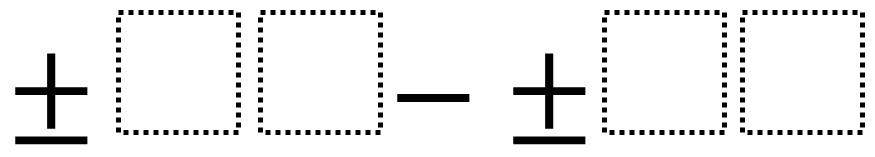Home > Grade 7 > Subtracting Two-Digit Numbers (Middle School)

# Subtracting Two-Digit Numbers (Middle School)

Directions: Make the smallest (or largest) difference by filling in the boxes using the whole numbers 1-9 no more than one time each### Hint

What does the number on the left represent?  What does the number on the right represent?

98 – 12 is the largest difference.  There are several answers for the smallest difference including 81-79.

Note: This problem’s difficulty can be adjusted by altering the number of digits (boxes), picking smallest or largest, or by picking either a positive, negative, or both.

Source: Robert Kaplinsky

## Converting a Fraction to a Decimal

Directions: Using the digits 1 to 9, at most one time each, place a digit …

1.We were just thinking of doing a question like this next week, but pretty simple. Subtract integers between -10 and +10, can’t use it twice, and make largest and smallest value possible.

Which is different than smallest difference I guess. Technically this question can make a negative…so your resulting “difference” would be a smaller value than 2?

And i just realized this is tagged as 1st, 2nd, and 7th grade, so it all depends on your limits.

But since I started this…is 80-79 (or anything like it) the smallest difference? A difference of 1?

Or can you do something like -97 – 86, and get -183? Would that be the smallest difference, or is that just a smaller value? Obviously depends on grade level…but at the 7th grade level, what’s the proper distinction? Is difference about distance apart on a number line, or is the resulting value the “difference”?

2.I have a similar question as to the addition problem like this one. Can’t I choose if a number is negative or positive? If I can, then what about 97 – (-86) as the largest difference?